# I think

I think a number. When I multiply it by five, and after that I subtract 477, I get the same number as if I multiplied it twice. What number do I think?

Result

x =  159

#### Solution:

5x - 477 = x*2

3x = 477

x = 159

Calculated by our simple equation calculator.

Leave us a comment of example and its solution (i.e. if it is still somewhat unclear...):Be the first to comment!#### To solve this verbal math problem are needed these knowledge from mathematics:

Do you have a linear equation or system of equations and looking for its solution? Or do you have quadratic equation?

## Next similar examples:

1. Number unknownAdela thought the two-digit number, she added it to its ten times and got 407. What number does she think?
2. Unknown number xyzFind the number that its triple is 24. Solve by equation.
3. Number of songs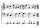Write an expression for the number of songs they need for this show. Evan and Peter have a radio show that has 2 parts. They need 4 fewer than 11 songs in the first part. In the second part, they need 5 fewer than 3 times the number of songs in the first p
4. What is missing (1000)What number is to add to get 1000?
5. Seven timesWhich number seven times is just as higher as 27, how much is smaller than 29?
6. Addition of Roman numbersAdded together and write as decimal number: LXVII + MLXIV
7. Expression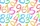If A=2 B=3 evaluate expression A(B+A) and multiply it by A
8. Farm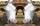Agricultural cooperative (farm) produced 14144 liters of milk for 16 days. Which exceeded the plan by 1984 liters. How many liters of milk a day had planned to produce?
9. Roman numeralsWrite numbers written in Roman numerals as decimal.
10. SummandOne of the summands is 145. The second is 10 more. Determine the sum of the summands.
11. School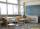27 students of 6.D class is going to a trip. They pay 9 € each. The teacher got 153 €. How many children have not paid?
12. Two numbers 6Fill two natural numbers a, b: 7 + blank- blank = 5
13. Six te 2If 3t-7=5t, then 6t=
14. Eq1Solve equation: 4(a-3)=3(2a-5)
15. If-then equationIf 5x - 17 = -x + 7, then x =
16. Hotel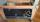The rooms in the mountain hotel are double and triple. Double rooms are 25 and triple are 17 more. How many rooms are there in this hotel?
17. Compare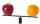Compare with characters >, <, =: 85.57 ? 80.83